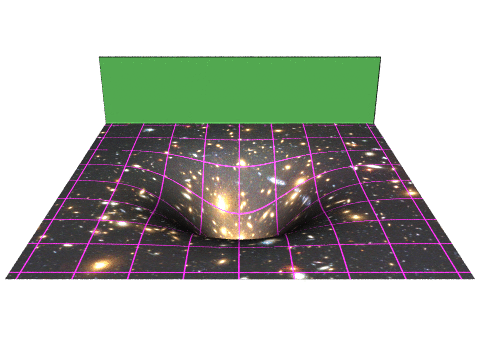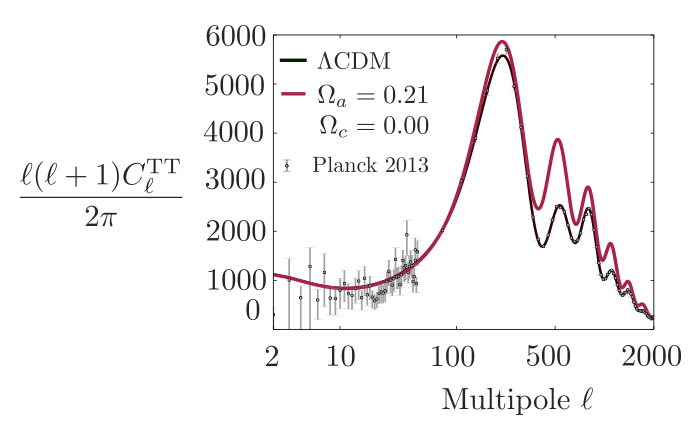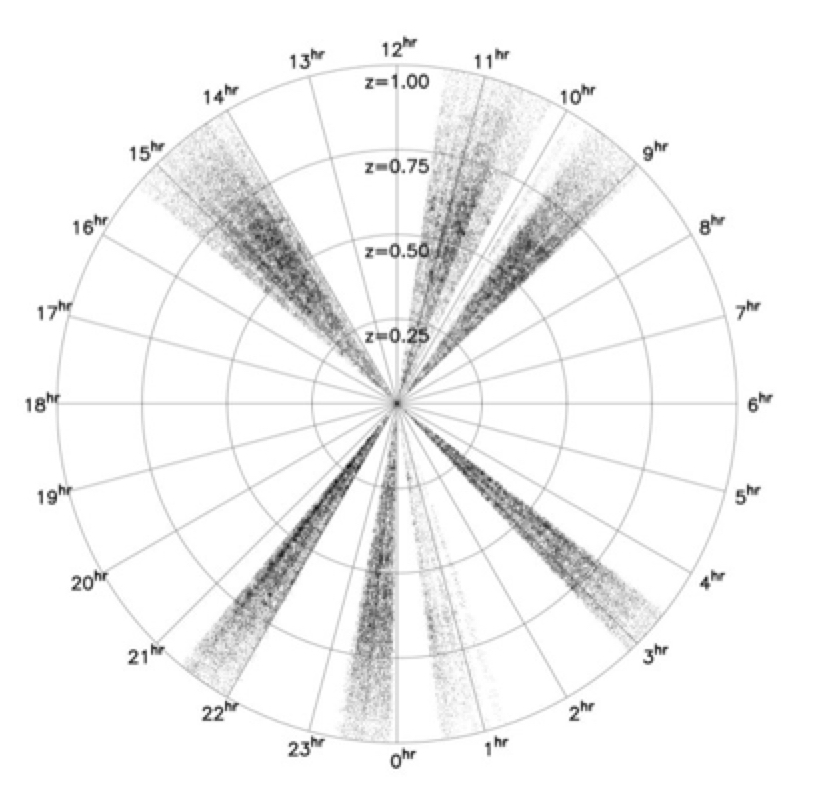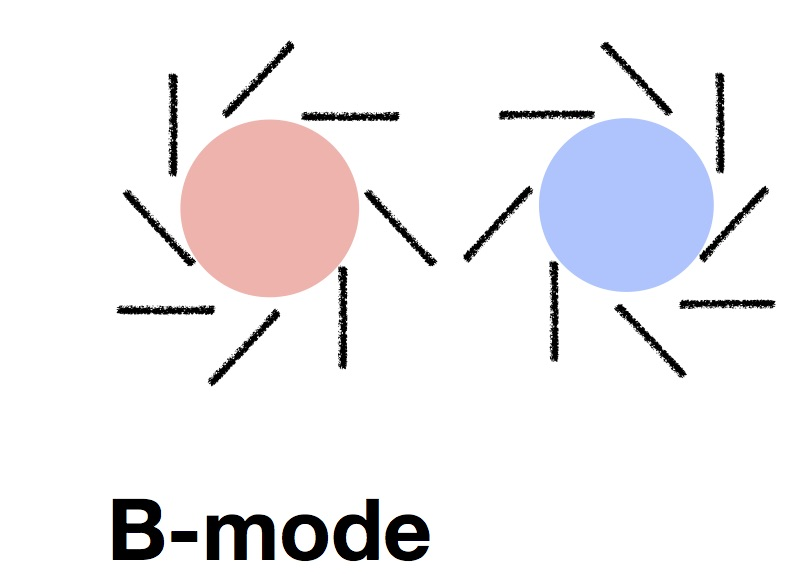# Ultra-light axions, the axiverse, and the energy scale of inflationAxions are a hypothetical new particle introduced to explain why there is not more charge-parity symmetry violation in the theory of the strong interaction. Depending on precise parameters, ultra-light axions ($m\leq 10^{-18}~{\rm eV}$) can act as dark matter or dark energy. For masses$m_{a} \leq 10^{-27}~{\rm eV}$, axions behave more like dark energy, while if$m_{a} \geq 10^{-27}~{\rm eV}$, axions behave more like dark matter. In this work, we created a new CMB anisotropy/galaxy clustering code called AxiCAMB, based on the Boltzmann code CAMB, which includes the effects of ultra-light axions. We then used this code to compare a wide suite of models including axions with precision cosmological data, and set new limits to ultra-light axions (shown above). In particular, if$10^{-32}~{\rm eV}\leq m_{a}\leq 10^{-26}~{\rm eV}$, axions can compose no more than$0.6 \%$ of the cosmological critical density. We also showed that a primordial gravitational wave detection at the level of current CMB limits would put ultra-light axion dark matter under extreme empirical pressure.

Collaborators: D. Marsh, R. Hlozek, and P. Ferreira.

Originally put forward as a solution to the strong CP problem, axions and axion-like particles (ALPs) (pseudo Nambu-Goldstones corresponding to a new U(1) symmetry) abound in string theory, where it is thought that ~600 axions may arise from the compactification of extra dimensions onto a Calubi-Yau manifold, spanning the mass range 10-33 eV< m <10-5 eV. This is the so-called axiverse. Because of weak gauge couplings, a free Peccei-Quinn Scale, an unpinned mislignment angle, and anharmonic effects in the axion potential, the axion density is best thought of as a free-parameter in these models, and the axion can satisfy cosmological density bounds easily at any mass. This picture is shown schematically by the figure below, where the potentials of several new complex scalars are shown, each with a corresponding pseudo Nambu-Goldstone boson.Ultra-light axions have several empirical consequences for cosmological observables, which we computed using AxiCAMB. The key physical effects are

• Suppression of structure on small scales, due to the macroscopic deBroglie wavelength of the axion. This would manifest itself as a suppression at small scales in the matter power spectrum, similar in effect to but different in origin from eV-keV mass neutrinos. Structure is suppressed beyond the axion “Jeans scale,”$k\gg k_{\rm J}\simeq \sqrt{m\mathcal{H}}$

This can be seen in the figure below, where the matter power spectrum is shown for CDM vs the most extreme axion dark matter models for effect. The take-away message of this figure is that the clustering of matter is suppressed on observable scales if the axion is too light or overly abundant.• For$m_{a}\leq 10^{-27}~{\rm eV}$, light axions act as a form of early dark energy, changing the distance to the surface of last scattering (where CMB photons come from) and changing the decay of gravitational potential wells at late times [an effect known as the late-time Integrated Sachs-Wolfe (or ISW) effect]. CMB photons pick up an additional temperature change as they pass through these decaying gravitational wells, as shown by the schematic below, reproduced from http://ifa.hawaii.edu/cosmowave/supervoids/the-integrated-sachs-wolfe-effect/.• The figure to the left shows the change in the CMB temperature anisotropy power spectrum as the axion density is changed for very low axion masses. The most dramatic change is the shift in acoustic peak locations due to CMB due to the altered angular diameter distance to the surface of last scattering. If other parameters are held fixed to restore this distance to its fiducial value, the remaining (large angular-scale or low$l$) effect is caused by the altered late-time ISW effect.• For$m_{a}\geq 10^{-27}~{\rm eV}$, axions begin behaving as matter before the end of the early-universe radiation dominated era, but change the growth of gravitational potential wells during the radiation dominated era (the early ISW effect). This affects the relative heights of the acoustic peaks in the CMB power spectrum, as shown below for a$10^{-32}~{\rm eV}$ axion.Using Planck 2013 temperature data and measurements of the galaxy correlation power spectrum from the WiggleZ survey (shown in the projected map below), we obtained the constraints shown at the top of this discussion. This problem turned out to be quite technically challenging due to the strange shape of the constrained region — we had to use the MultiNest algorithm (which explores parameter spaces with multiple likelihood peaks efficiently) in order to obtain our constraints. Read  arXiv:1410.2896 (Phys. Rev. D 91, 103512) for details!The global symmetry associated with axions is typically (in most theoretical scenarios) broken at high energy scales ($10^{14}~{\rm GeV} ). If inflation happens at high energy scales, the axion field picks up quantum fluctuations associated with the deSitter horizon of the universe during inflation. These later become dark matter (or dark energy) density fluctuations, offset from fluctuations in relativistic species and baryons. Due to this offset, these are `entropy’ or isocurvature fluctuations that supplement the dominant inflationary adiabatic fluctuations, illustrated by the attached schematic, which also shows the resulting adiabatic and isocurvature CMB temperature power spectra [Note that the precise shape of the axion isocurvature power spectrum depends on the axion mass]. CDM denotes cold dark matter below. The axion isocurvature power spectra are computed using AxiCAMB.CMB temperature power spectra measured by the Planck satellite constrain the fractional isocurvature power (in axion-type isocurvature, which is uncorrelated with the dominant adiabatic fluctuations) to be$4\%$, imposing in turn the upper limit$\frac{\Omega_{a}}{\Omega_{a}+\Omega_{c}}\leq 10^{-12} \left(\frac{10^{14}~{\rm GeV}}{H_{I}}\right)^{7/2}$

to the fractional energy density of standard strong-interaction motivated axions, where$\Omega_{c}$ is the energy density (relative to the current critical density of the universe) of standard cold dark matter (CDM) and$H_{I}$ is the Hubble parameter during inflation, which sets the amplitude of vacuum fluctuations in all fields present during that era. For ultra-light axions with no connection to the QCD phase transition, the limit is more permissive but still quite restrictive:$\frac{\Omega_{a}}{\Omega_{a}+\Omega_{c}}\leq 10^{-3} \left(\frac{10^{14}~{\rm GeV}}{H_{I}}\right)^{7/2}$.

The inflationary energy scale sets the amplitude of primordial gravitational waves, which in turn would imprint a curl (or B-type) pattern on CMB polarization anisotropies, as shown in the below schematic. In other words, if current upper limits ($H_{I}\sim 10^{14}~{\rm GeV}$) from BICEP2/Keck and similar experiments are saturated by a detection, standard and ultra-light axions are in trouble. The details are discussed in arXiv:1403.4216 (Phys. Rev. Lett.113.011801), arXiv: 1303.3008 (Phys. Rev. D 87.121701).